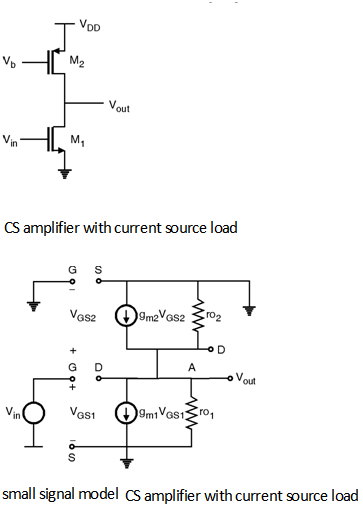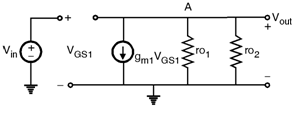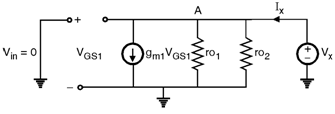Home > Analog CMOS Design > MOSFET Amplifiers > CS Amplifier with Current Source Load

CS Amplifier with Current Source Load :

Figure below shows the circuit diagram of CS amplifier with current source load. In this a current source is made by using a PMOS transistor operated in saturation mode by using a Gate bias Vb. The small signal model of this circuit is also shown in Figure belowThe equivalent circuit can be drawn as shown in figure belowBy applying KVL, Vin = VGS1

By applying KCL at node A

gm1 VGS1 + + = 0

\ gm1 Vin = - Vout

\ = An = - gm1 (ro1 || ro2)

In order to derive the output impedance consider the circuit shown in Figure below.\ By applying KCL at node A,

We get, gm1 VGS1 + + = Ix

Vx = Ix [ VGS1 = 0]

\ = Rout =

\ Rout = (ro1 || ro2)

\ Hence the output impedance of current source load of CS amplifier is ro 1 || ro2.